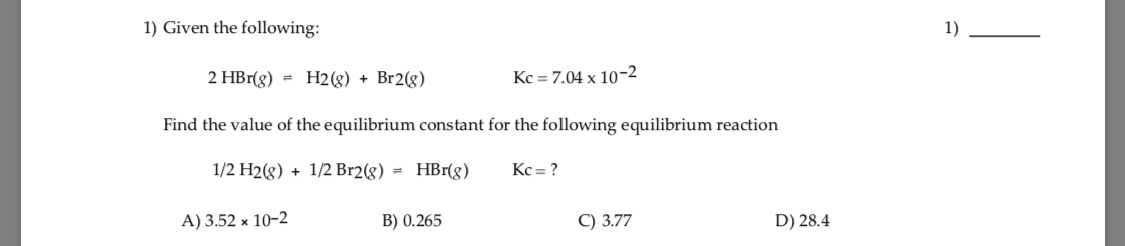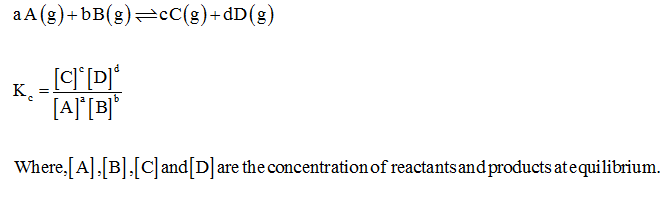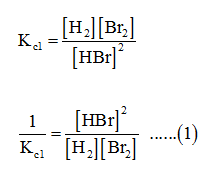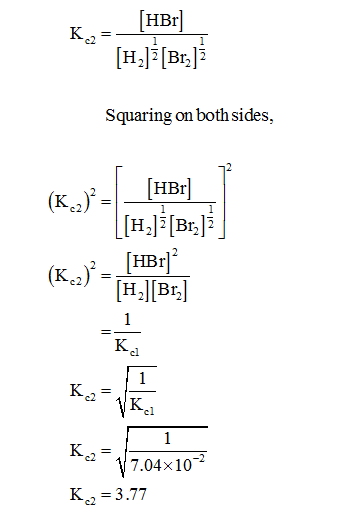# 1) Given the following:1)2 HBr(g) = H2(g) + Br2(g)Kc = 7.04 x 10-2Find the value of the equilibrium constant for the following equilibrium reaction1/2 H2(g) + 1/2 Br2(g) = HBr(8)Kc = ?A) 3.52 x 10-2B) 0.265C) 3.77D) 28.4

Question
6 viewshelp_outlineImage Transcriptionclose1) Given the following: 1) 2 HBr(g) = H2(g) + Br2(g) Kc = 7.04 x 10-2 Find the value of the equilibrium constant for the following equilibrium reaction 1/2 H2(g) + 1/2 Br2(g) = HBr(8) Kc = ? A) 3.52 x 10-2 B) 0.265 C) 3.77 D) 28.4 fullscreen
check_circle

Step 1

The equilibrium constant, Kc for a reaction is given as :Step 2

The given reaction is :

2 HBr (g) ↔ H2 (g) + Br2 (g)          Kc1 = 7.04 × 10-2

The equilibrium constant, Kc1 for this reaction can be expressed as :Step 3

Again, the given reaction is :

½ H2 (g) + ½ Br2 (g) ↔ HBr (g)          Kc2 = ?

The equilibrium constant, Kc2 for this reaction can be expressed as :...

### Want to see the full answer?

See Solution

#### Want to see this answer and more?

Solutions are written by subject experts who are available 24/7. Questions are typically answered within 1 hour.*

See Solution
*Response times may vary by subject and question.
Tagged in